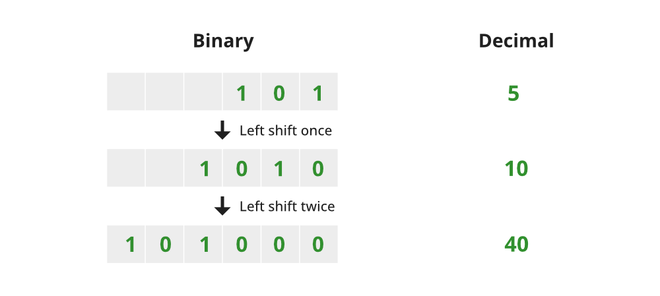# Left Shift Operator in Java

• Difficulty Level : Basic
• Last Updated : 28 Dec, 2021

The decimal representation of a number is a base-9 number system having only nine states as 0, 1, 2, 3, 4, 5, 6, 7, 8, and 9. For example, 4, 10, 16, etc.

The Binary representation of a number is a base-2 number system having only two states as 0 and 1. For example, the binary representation of 4, a base-9 decimal number system is given by 100, 10 as 1010, 16 as 1000, etc.

### Left Shift

The left shift means that shift each of the bits in binary representation toward the left. For example, when we say left shift 5 or 101 by one position. We will shift each of the bits by one position towards the left. So after shifting the number 5 towards left by one position, the number obtained is 10 or 1010. Now let us again left shift 10 by two positions. Again, we will shift each of the bits by two positions towards the left. The number obtained is 40 or 101000.

Note: Left shifting a number by certain positions is equivalent to multiplying the number by two raised to the power of the specified positions. That is,

`left shift x by n positions <=> x * 2n `### Left Shift Operator in Java

Most of the languages provide left shift operator using which we can left shift a number by certain positions and Java is one of them. The syntax of the left-shift operator in Java is given below,

Syntax:

```x << n

Here,
x: an integer
n: a non-negative integer ```

Return type: An integer after shifting x by n positions toward left

Exception: When n is negative the output is undefined

Below is the program to illustrate how we can use the left shift operator in Java.

Example 1:

## Java

 `// Java program to illustrate the ``// working of left shift operator`` ` `import` `java.io.*;`` ` `class` `GFG {``   ` `      ``// Main method``    ``public` `static` `void` `main (String[] args) {``         ` `        ``// Number to be shifted``        ``int` `x = ``5``;``           ` `        ``// Number of positions ``        ``int` `n = ``1``;``         ` `        ``// Shifting x by n positions towards left using left shift operator``        ``int` `answer = x << n;``         ` `        ``// Print the number obtained after shifting x by n positions towards left``        ``System.out.println(``"Left shift "` `+ x + ``" by "` `+ n + ``" positions : "` `+ answer);``         ` `        ``// Number to be shifted``        ``x = answer;``           ` `        ``// Number of positions ``        ``n = ``2``;``         ` `        ``// Shifting x by n positions towards left using left shift operator``        ``answer = answer << n;``           ` `        ``// Print the number obtained after shifting x by n positions towards left``        ``System.out.println(``"Left shift "` `+ x + ``" by "` `+ n + ``" positions : "` `+ answer);``         ` `    ``}``}`
Output
```Left shift 5 by 1 positions : 10
Left shift 10 by 2 positions : 40```

Example 2:

## Java

 `// Java program to illustrate the ``// working of left shift operator`` ` `import` `java.io.*;`` ` `class` `GFG {``   ` `      ``// Main method``    ``public` `static` `void` `main (String[] args) {``         ` `        ``// Number to be shifted``        ``int` `x = -``2``;``           ` `        ``// Number of positions ``        ``int` `n = ``1``;``         ` `        ``// Shifting x by n positions towards ``        ``// left using left shift operator``        ``int` `answer = x << n;``         ` `        ``// Print the number obtained after shifting x by n positions towards left``        ``System.out.println(``"Left shift "` `+ x + ``" by "` `+ n + ``" positions : "` `+ answer);``         ` `        ``// Number to be shifted``        ``x = answer;``           ` `        ``// Number of positions ``        ``n = ``2``;``         ` `        ``// Shifting x by n positions towards``        ``// left using left shift operator``        ``answer = answer << n;``           ` `        ``// Print the number obtained after shifting x by n positions towards left``        ``System.out.println(``"Left shift "` `+ x + ``" by "` `+ n + ``" positions : "` `+ answer);``         ` `    ``}``}`
Output
```Left shift -2 by 1 positions : -4
Left shift -4 by 2 positions : -16```

Note: For arithmetic left shift, since filling the right-most vacant bits with 0s will not affect the sign of the number, the vacant bits will always be filled with 0s, and the sign bit is not considered. Thus, it behaves in a way identical to the logical (unsigned) left shift. So there is no need for a separate unsigned left sift operator.

My Personal Notes arrow_drop_up